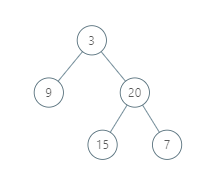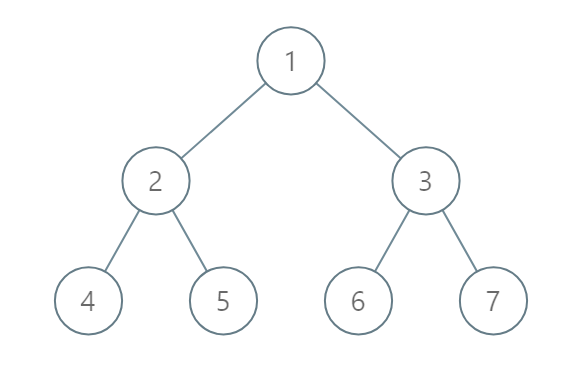Formatted question description: https://leetcode.ca/all/987.html

# 987. Vertical Order Traversal of a Binary Tree (Medium)

Given a binary tree, return the vertical order traversal of its nodes values.

For each node at position (X, Y), its left and right children respectively will be at positions (X-1, Y-1) and (X+1, Y-1).

Running a vertical line from X = -infinity to X = +infinity, whenever the vertical line touches some nodes, we report the values of the nodes in order from top to bottom (decreasing Y coordinates).

If two nodes have the same position, then the value of the node that is reported first is the value that is smaller.

Return an list of non-empty reports in order of X coordinate.  Every report will have a list of values of nodes.

Example 1:Input: [3,9,20,null,null,15,7]
Output: [,[3,15],,]
Explanation:
Without loss of generality, we can assume the root node is at position (0, 0):
Then, the node with value 9 occurs at position (-1, -1);
The nodes with values 3 and 15 occur at positions (0, 0) and (0, -2);
The node with value 20 occurs at position (1, -1);
The node with value 7 occurs at position (2, -2).


Example 2:Input: [1,2,3,4,5,6,7]
Output: [,,[1,5,6],,]
Explanation:
The node with value 5 and the node with value 6 have the same position according to the given scheme.
However, in the report "[1,5,6]", the node value of 5 comes first since 5 is smaller than 6.


Note:

1. The tree will have between 1 and 1000 nodes.
2. Each node's value will be between 0 and 1000.

Related Topics:
Hash Table, Tree

## Solution 1.

Use a map<int, map<int, multiset<int>>> m to store the values – m[node->x][node->y].insert(node->val). (Using set instead of multiset can also pass this problem. I guess LeetCode uses the node values as IDs and assumes the uniqueness of the values. I used multiset here to be safe.)

In this way, the values are sorted first in asending order of the x values, then in asending order of y values, then in asending order of node values.

Note that we shouldn’t sort the values with the same X values all together, we should only sort them if they have the same position, i.e. when both their x and y values are equal.

// OJ: https://leetcode.com/problems/vertical-order-traversal-of-a-binary-tree/

// Time: O(NlogN)
// Space: O(N)
class Solution {
map<int, map<int, multiset<int>>> m;
void dfs(TreeNode *root, int x, int y) {
if (!root) return;
m[x][y].insert(root->val);
dfs(root->left, x - 1, y + 1);
dfs(root->right, x + 1, y + 1);
}
public:
vector<vector<int>> verticalTraversal(TreeNode* root) {
dfs(root, 0, 0);
vector<vector<int>> ans;
for (auto &[x, mm] : m) {
ans.emplace_back();
for (auto &[y, vals] : mm) {
for (int n : vals) ans.back().push_back(n);
}
}
return ans;
}
};


Java

/**
* Definition for a binary tree node.
* public class TreeNode {
*     int val;
*     TreeNode left;
*     TreeNode right;
*     TreeNode(int x) { val = x; }
* }
*/
class Solution {
public List<List<Integer>> verticalTraversal(TreeNode root) {
List<List<Integer>> verticalTraversal = new ArrayList<List<Integer>>();
if (root == null)
return verticalTraversal;
Queue<TreeNode> queue = new LinkedList<TreeNode>();
Queue<Struct> structQueue = new LinkedList<Struct>();
queue.offer(root);
Struct rootStruct = new Struct(0, 0, root.val);
structQueue.offer(rootStruct);
List<Struct> structList = new ArrayList<Struct>();
while (!queue.isEmpty()) {
TreeNode node = queue.poll();
Struct nodeStruct = structQueue.poll();
int position = nodeStruct.getPosition();
int depth = nodeStruct.getDepth();
TreeNode left = node.left;
TreeNode right = node.right;
if (left != null) {
queue.offer(left);
Struct leftStruct = new Struct(position - 1, depth + 1, left.val);
structQueue.offer(leftStruct);
}
if (right != null) {
queue.offer(right);
Struct rightStruct = new Struct(position + 1, depth + 1, right.val);
structQueue.offer(rightStruct);
}
}
Collections.sort(structList);
int size = structList.size();
int index = 0;
int prevPosition = Integer.MAX_VALUE;
while (index < size) {
Struct struct = structList.get(index);
int position = struct.getPosition();
if (position == prevPosition) {
int totalSize = verticalTraversal.size();
List<Integer> previousList = verticalTraversal.remove(totalSize - 1);
} else {
List<Integer> currentList = new ArrayList<Integer>();
}
prevPosition = position;
index++;
}
return verticalTraversal;
}
}

class Struct implements Comparable<Struct> {
private int position;
private int depth;
private int value;

public Struct() {

}

public Struct(int position, int depth, int value) {
this.position = position;
this.depth = depth;
this.value = value;
}

public int compareTo(Struct struct2) {
if (this.position != struct2.position)
return this.position - struct2.position;
else if (this.depth != struct2.depth)
return this.depth - struct2.depth;
else
return this.value - struct2.value;
}

public int getPosition() {
return position;
}

public int getDepth() {
return depth;
}

public int getValue() {
return value;
}
}